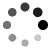[原创]Codeforces #353 div.2 Infinite Sequence&Restoring Painting&Money Transfers前三题题解

2016-05-18 21:59:54 Tabris_ 阅读数：531

https://blog.csdn.net/qq_33184171/article/details/51447994

## A

A. Infinite Sequence
time limit per test1 second
memory limit per test256 megabytes
inputstandard input
outputstandard output
Vasya likes everything infinite. Now he is studying the properties of a sequence s, such that its first element is equal to a (s1 = a), and the difference between any two neighbouring elements is equal to c (si - si - 1 = c). In particular, Vasya wonders if his favourite integer b appears in this sequence, that is, there exists a positive integer i, such that si = b. Of course, you are the person he asks for a help.

Input
The first line of the input contain three integers a, b and c ( - 109 ≤ a, b, c ≤ 109) — the first element of the sequence, Vasya’s favorite number and the difference between any two neighbouring elements of the sequence, respectively.

Output
If b appears in the sequence s print “YES” (without quotes), otherwise print “NO” (without quotes).

Examples

input
1 7 3
output
YES

input
10 10 0
output
YES

input
1 -4 5
output
NO

input
0 60 50
output
NO

Note
In the first sample, the sequence starts from integers 1, 4, 7, so 7 is its element.

In the second sample, the favorite integer of Vasya is equal to the first element of the sequence.

In the third sample all elements of the sequence are greater than Vasya’s favorite integer.

In the fourth sample, the sequence starts from 0, 50, 100, and all the following elements are greater than Vasya’s favorite integer.

## B

B. Restoring Painting
time limit per test1 second
memory limit per test256 megabytes
inputstandard input
outputstandard output
Vasya works as a watchman in the gallery. Unfortunately, one of the most expensive paintings was stolen while he was on duty. He doesn’t want to be fired, so he has to quickly restore the painting. He remembers some facts about it.

The painting is a square 3 × 3, each cell contains a single integer from 1 to n, and different cells may contain either different or equal integers.
The sum of integers in each of four squares 2 × 2 is equal to the sum of integers in the top left square 2 × 2.
Four elements a, b, c and d are known and are located as shown on the picture below.Help Vasya find out the number of distinct squares the satisfy all the conditions above. Note, that this number may be equal to 0, meaning Vasya remembers something wrong.

Two squares are considered to be different, if there exists a cell that contains two different integers in different squares.

Input
The first line of the input contains five integers n, a, b, c and d (1 ≤ n ≤ 100 000, 1 ≤ a, b, c, d ≤ n) — maximum possible value of an integer in the cell and four integers that Vasya remembers.

Output
Print one integer — the number of distinct valid squares.

Examples
input
2 1 1 1 2
output
2
input
3 3 1 2 3
output
6
Note
Below are all the possible paintings for the first sample.In the second sample, only paintings displayed below satisfy all the rules.TablesAreCool
x1ax2
b??c
x3dx4

2x2的情况为
1: x1+a+b+??
2: x2+a+c+??
3: x3+d+b+??
4: x4+c+d+??

x1+1,x2+1,x3+1,x4+1 也满足题意 （当中最大值<=n）

a+b, b+d,d+c,a+c的最大值 这时候其对应的x值为1

count（maxX~n) * n;

## C

C. Money Transfers
time limit per test1 second
memory limit per test256 megabytes
inputstandard input
outputstandard output
There are n banks in the city where Vasya lives, they are located in a circle, such that any two banks are neighbouring if their indices differ by no more than 1. Also, bank 1 and bank n are neighbours if n > 1. No bank is a neighbour of itself.

Vasya has an account in each bank. Its balance may be negative, meaning Vasya owes some money to this bank.

There is only one type of operations available: transfer some amount of money from any bank to account in any neighbouring bank. There are no restrictions on the size of the sum being transferred or balance requirements to perform this operation.

Vasya doesn’t like to deal with large numbers, so he asks you to determine the minimum number of operations required to change the balance of each bank account to zero. It’s guaranteed, that this is possible to achieve, that is, the total balance of Vasya in all banks is equal to zero.

Input
The first line of the input contains a single integer n (1 ≤ n ≤ 100 000) — the number of banks.

The second line contains n integers ai ( - 109 ≤ ai ≤ 109), the i-th of them is equal to the initial balance of the account in the i-th bank. It’s guaranteed that the sum of all ai is equal to 0.

Output
Print the minimum number of operations required to change balance in each bank to zero.

Examples

input
3
5 0 -5
output
1

input
4
-1 0 1 0
output
2

input
4
1 2 3 -6
output
3

Note
In the first sample, Vasya may transfer 5 from the first bank to the third.

In the second sample, Vasya may first transfer 1 from the third bank to the second, and then 1 from the second to the first.

In the third sample, the following sequence provides the optimal answer:

transfer 1 from the first bank to the second bank;
transfer 3 from the second bank to the third;
transfer 6 from the third bank to the fourth.

（略带意淫的翻译）

（区间和为0的充要条件是区间两端点的前缀和相等即mp[si] == mp[sj]）那么这道题就做出来了